# The SIMLIN Procedure

### Dynamic Multipliers

For models that have only first-order lags, the equation of the reduced form of the system can be rewritten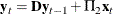D is a matrix formed from the columns of
plus some columns of zeros, arranged in the order in which the variables meet the lags. The elements ofare called impact multipliers because they show the immediate effect of changes in each exogenous variable on the values of the endogenous variables. This equation can be rewritten as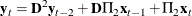The matrix formed by the product Dshows the effect of the exogenous variables one lag back; the elements in this matrix are called interim multipliers and are computed and printed when the INTERIM= option is specified in the PROC SIMLIN statement. The i th period interim multipliers are formed by D.

The series can be expanded as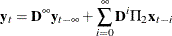A permanent and constant setting of a value for x has the following cumulative effect: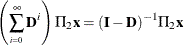The elements of (I-D)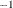are called the total multipliers. Assuming that the sum converges and that (I-D ) is invertible, PROC SIMLIN computes the total multipliers when the TOTAL option is specified in the PROC SIMLIN statement.